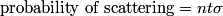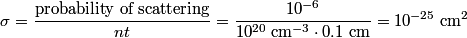## Solution to 1986 Problem 42

 The relevant equation is\begin{align*}\mbox{probability of scattering} = n t \sigma\end{align*}where$n$ is the number density of targets,$t$ is the thickness of the target, and$\sigma$ is the cross section.\begin{align*}\sigma = \frac{\mbox{probability of scattering}}{n t} = \frac{10^{-6}}{10^{20} \;\mathrm{cm}^{-3} \cdot 0.1 \mb...Therefore, answer (C) is correct.